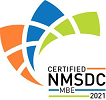Red Comet## Course Details:

This course covers the important topics necessary before a student takes on a full-fledged Algebra course. Student learns about monomials, polynomials, expressions and their properties. The course teaches how to solve for one-variable expressions. They also learn about analyzing data and making inferences based on different types of graphs.

## Syllabus:

Section 1: Monomials
• Identifying Monomials
• Multiplying Monomials
• Dividing Monomials
• More Multiplication and Division with Monomials
• Powers of Monomials
• Word Problems
Section 2: Polynomials
• Introduction to Polynomials
• Addition and Subtraction with Polynomials
• Adding Polynomials to find the Perimeter
• Multiply a Polynomial by a Monomial
• Multiplying Two Binomials
• Multiplying Two Binomials: Special Cases
• Multiplying Polynomials
• Word Problems
Section 3: Expressions and Properties
• Writing Variable Expressions
• Writing Variable Expressions from Diagrams
• Writing Variable Expressions from Word Problems
• Evaluating One-Variable Expressions
• Evaluating Multi-Variable Expressions
• Identifying Terms and Coefficients
• Properties of Addition and Multiplication
• Distributive Property
• Equivalent Expressions
• Adding and Subtracting Like Terms
• Word Problems
Section 4: Equations with Variables
• Solving for x in an equation
• Writing an Equation from Word Problems
• Writing and Solving Equations with Diagrams
• Properties of Equalities
• Solving Two-Step Equations
• Solving Multi-Step Equations
• Solving Equations Involving Like Terms
• Solving Equations with Variables on Both Sides
• Word Problems
Section 5: Data and Graphs
• Interpreting Tables
• Interpreting Bar Graphs
• Creating Bar Graphs
• Interpreting Line Graphs
• Creating Line Graphs
• Interpreting Line Plots
• Creating Line Plots
• Creating and Interpreting Line Plots with Fractions
• Interpreting Stem and Leaf Plots
• Interpreting Histograms
• Creating Histograms
• Creating Frequency Charts
• Interpreting Blocks and Whisper Plots
• Scatter Plots
• Interpreting Circle Graphs
• Circle Graphs and Central Angles
• Choosing the best type of Graph
• Word Problems

### Accreditation & Approvals